2019-11-28 21:00
827

# 使用 TensorFlow 机器学习自动化 RF 侧信道攻击

## 1.概述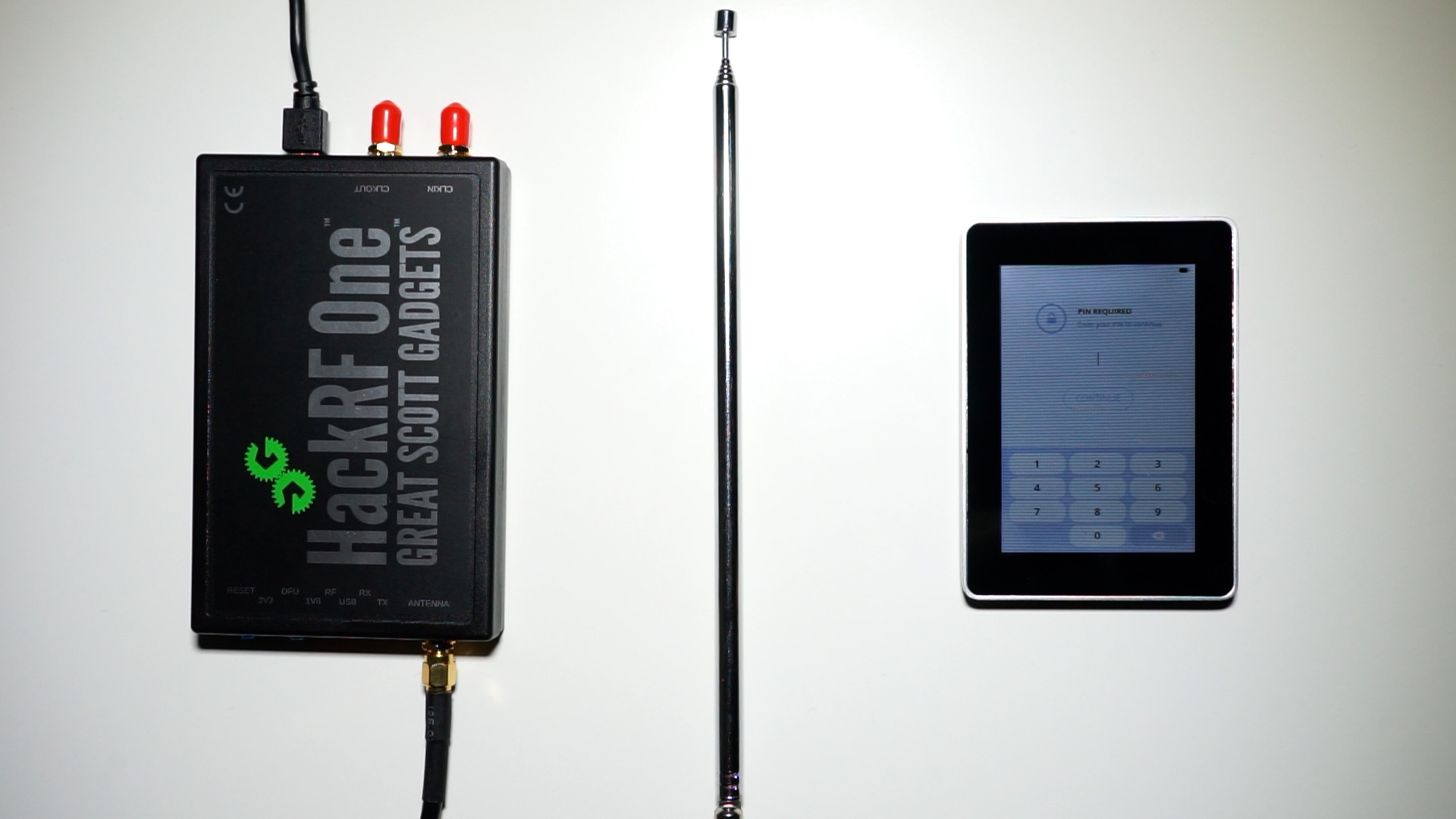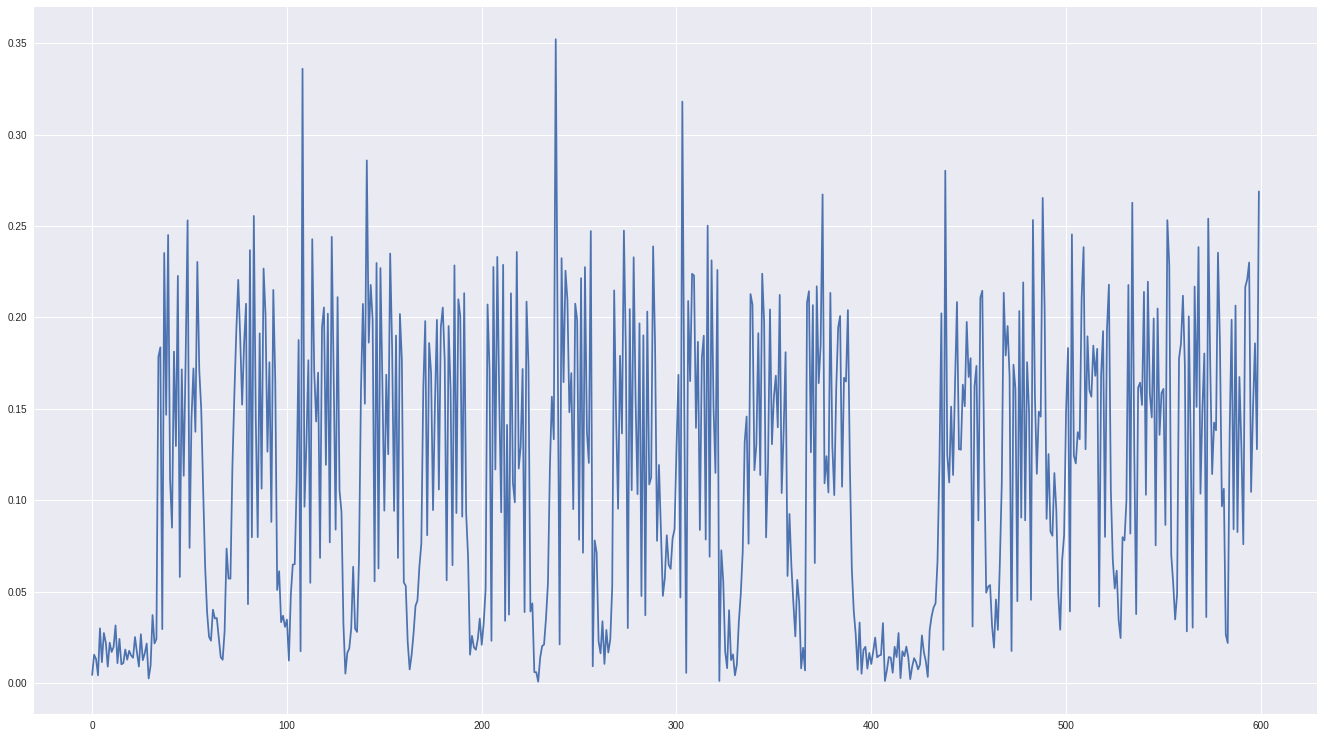## 2.收集训练数据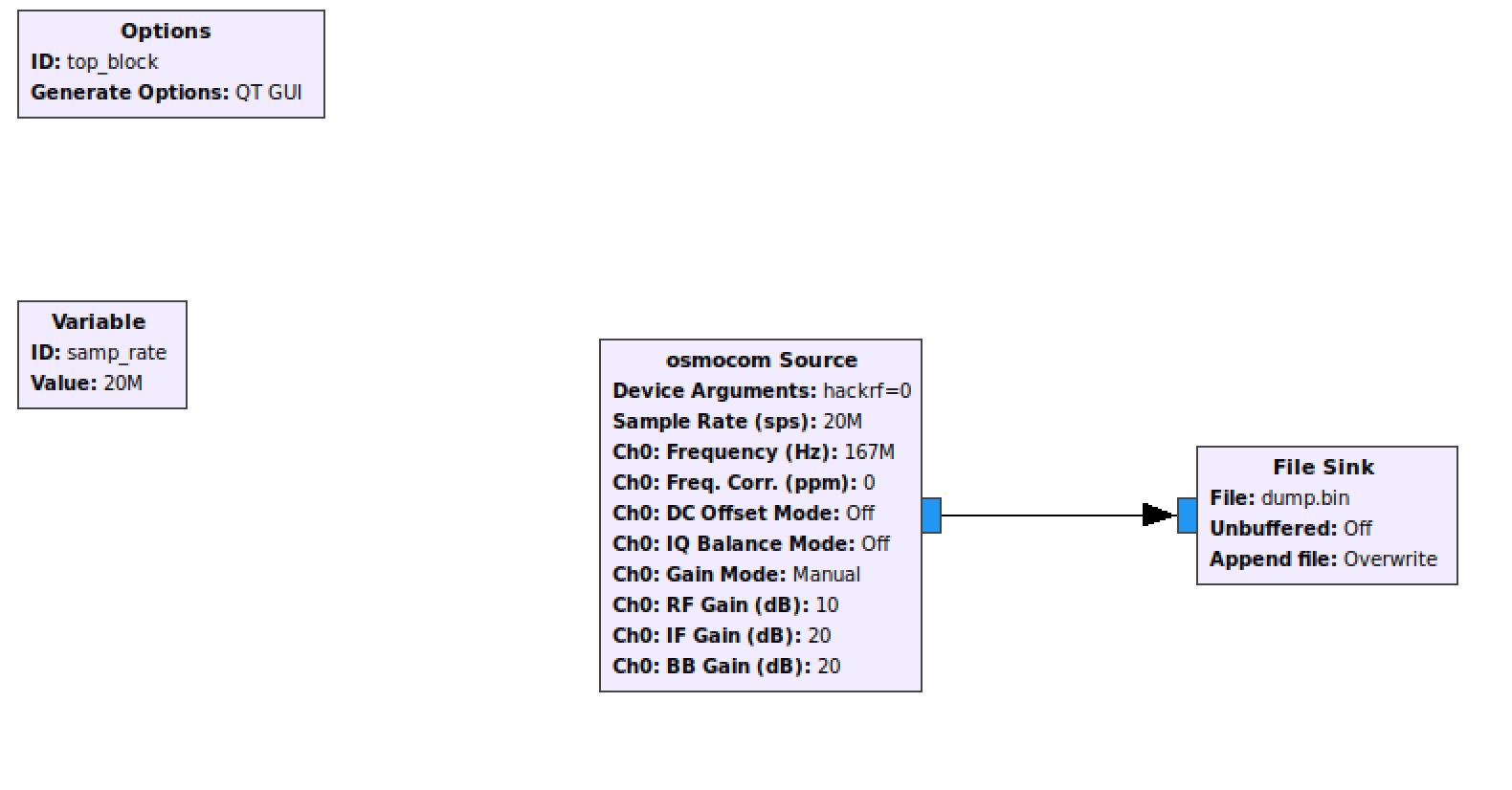## 3.准备 TensorFlow 的数据

```data0 = np.load("try_0_1_short.npy")[:data_length]
plt.figure()
plt.plot(data0.real)
plt.plot(np.abs(data0))
plt.show()
```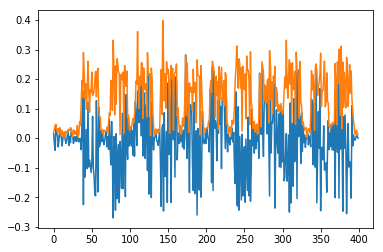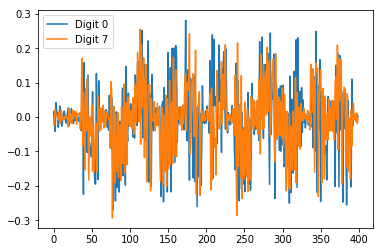```# Configure the bandpass for the signal
bandpass_start = 0.55
bandpass_end = 0.60
bandpass_order = 4

def apply_bandpass(s, order=bandpass_order, low=bandpass_start, high=bandpass_end):
#     butter(order, [low, high], btype='band')
b, a = signal.butter(order, [low, high], btype='band')
zi = signal.lfilter_zi(b, a)
z, _ = signal.lfilter(b, a, s, zi=zi*s)
return z
```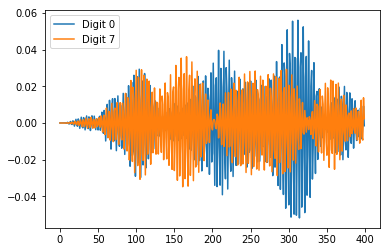## 4.加载，标记，分组

• 训练数据集：用于训练神经网络。
• 测试数据集：用于在所有的训练完成、神经网络参数确定后，验证神经网络的可靠性。

• 分别从各个样本文件加载 40 个 I/Q 样本
• 带通滤波处理
• 取滤波后信号的绝对值
• 把数据转换成 20x20 的矩阵（便于后面的可视化处理）
• 用 MinMaxScaler 归一化到 0.0 与 1.0 之间
• 把数据、数据的标记（本案例中即信号代表的按键数字）放入两个数组中
• 打乱每对数据（数据本身+数据标记）在两个数组内的顺序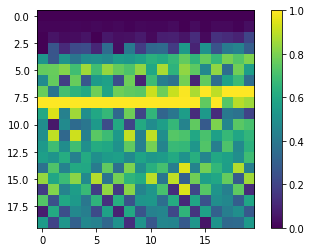## 5.构建神经网络

• Flatten layer：把平面的二维数组转换成一维
• Dense layer：128 路输出的层，使用 Rectified Linear Unit（ReLU）激活函数
• Dense layer：10 路输出的层（1 位数字 1 路输出），使用 SoftMax 激活函数

```model = keras.Sequential([
keras.layers.Flatten(input_shape=(20, 20)),
keras.layers.Dense(128, activation=tf.nn.relu),
keras.layers.Dense(10, activation=tf.nn.softmax)
])
```

```model.compile(optimizer=tf.train.AdamOptimizer(),
loss='sparse_categorical_crossentropy',
metrics=['accuracy'])
```

## 6.训练神经网络

```history = model.fit(train_data, train_labels, epochs=200, verbose=0, validation_split=0.2)
```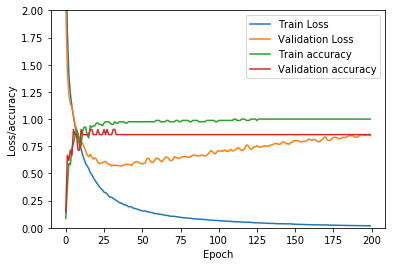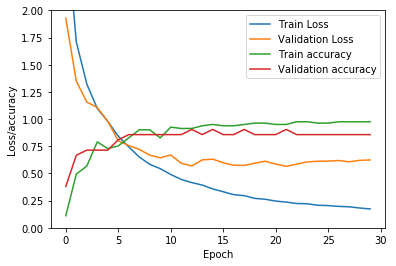## 7.测试神经网络

```test_loss, test_acc = model.evaluate(test_data, test_labels)
print('Accuracy on testdata:', test_acc * 100, "%")
```
```26/26 [==============================] - 0s 58us/sample - loss: 0.1962 - acc: 0.9615
Accuracy on testdata: 96.15384340286255 %
```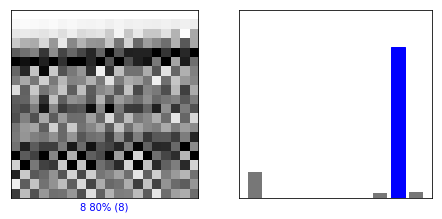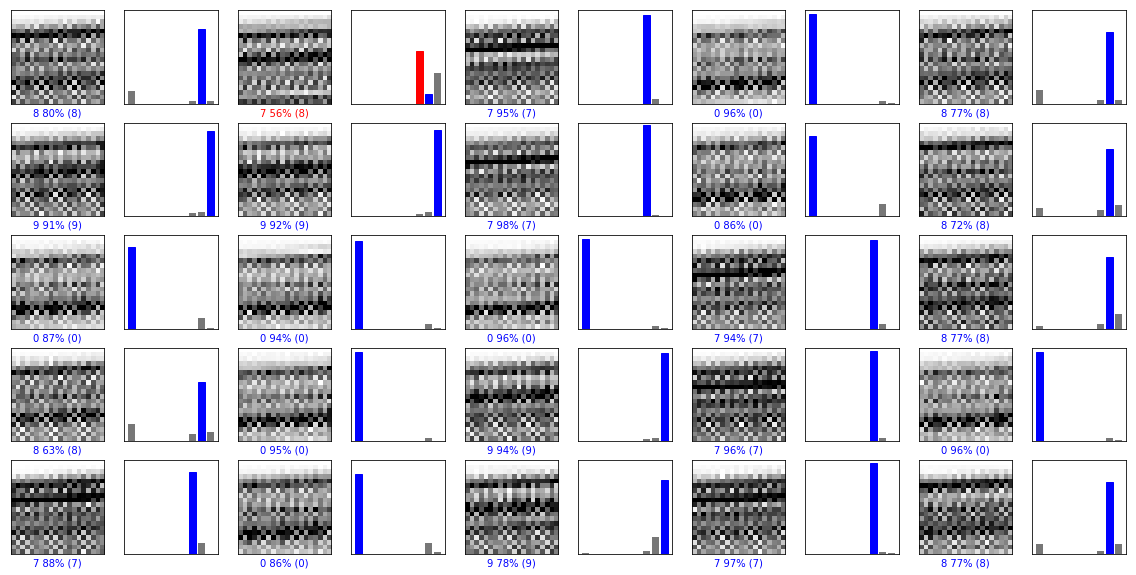## 8.总结

[公告]安全测试和项目外包请将项目需求发到看雪企服平台：https://qifu.kanxue.com

4

12019-11-28 22:06
2
02019-11-28 22:21
3
03 2019-11-29 08:05
4
03 2019-11-29 08:05
5
02019-11-29 09:24
6
03 2019-11-29 09:43
7
0xiaokangpwn 老板图又挂了，麻烦再修修图啊